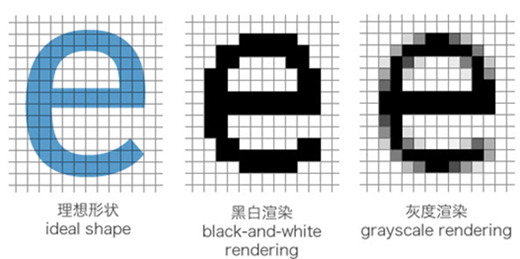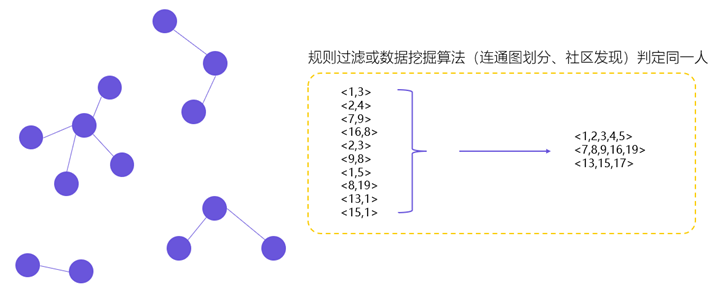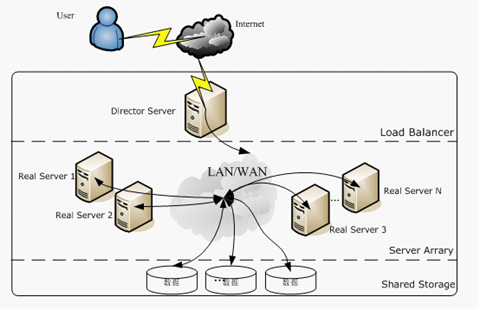机器学习聚类算法之层次聚类

层次聚类简介

• Divisive 层次聚类：又称自顶向下（top-down）的层次聚类，最开始所有的对象均属于一个cluster，每次按一定的准则将某个cluster 划分为多个cluster，如此往复，直至每个对象均是一个cluster。
• Agglomerative 层次聚类：又称自底向上（bottom-up）的层次聚类，每一个对象最开始都是一个cluster，每次按一定的准则将最相近的两个cluster合并生成一个新的cluster，如此往复，直至最终所有的对象都属于一个cluster。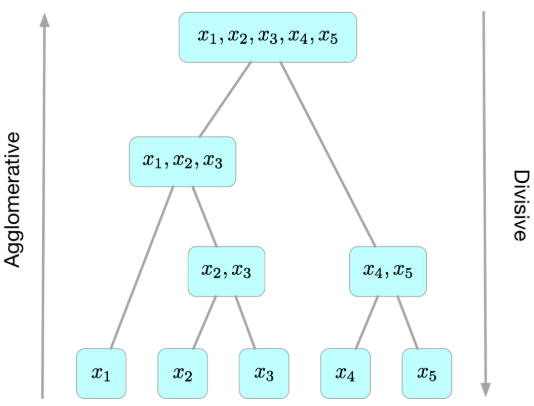自顶向下的层次聚类算法(Divisive)

Hierarchical K-means算法

Hierarchical K-means算法是“自顶向下”的层次聚类算法，用到了基于划分的聚类算法那K-means，算法思路如下：

• 首先，把原始数据集放到一个簇C，这个簇形成了层次结构的最顶层
• 使用K-means算法把簇C划分成指定的K个子簇$C_i, i=1,2,…,k$，形成一个新的层
• 对于步骤2所生成的K个簇，递归使用K-means算法划分成更小的子簇，直到每个簇不能再划分（只包含一个数据对象）或者满足设定的终止条件。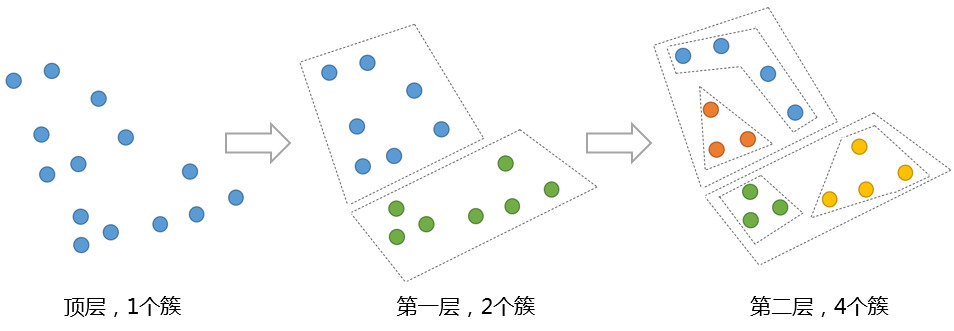Hierarchical K-means算法一个很大的问题是，一旦两个点在最开始被划分到了不同的簇，即使这两个点距离很近，在后面的过程中也不会被聚类到一起。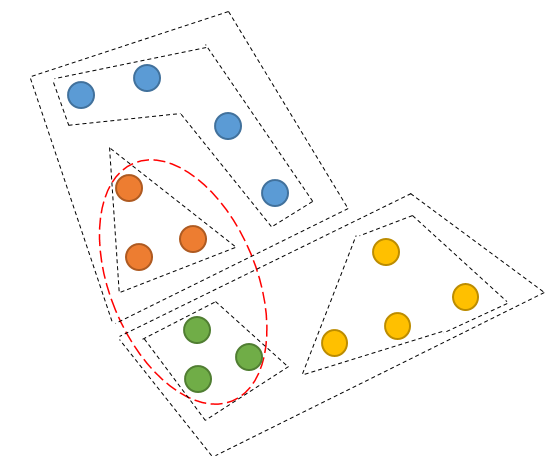Bisecting k-means聚类算法，即二分k均值算法，是分层聚类（Hierarchical clustering）的一种。更多关于二分k均值法，可以查看聚类算法之K-Means

自底向上的层次聚类算法(Agglomerative)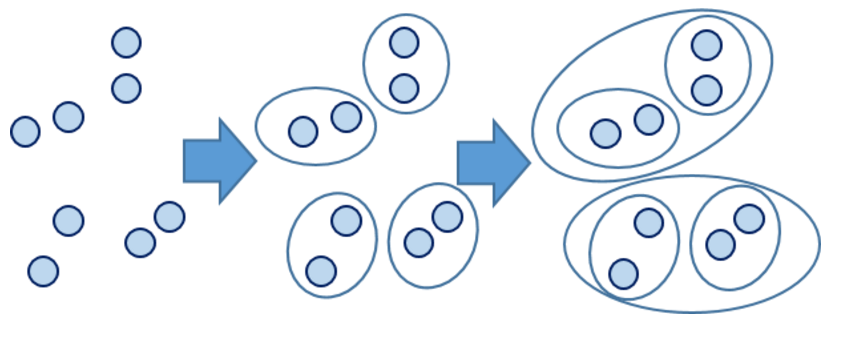Agglomerative算法示例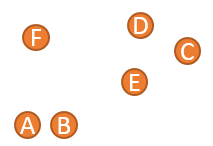1、 将A到F六个点，分别生成6个簇
2、 找到当前簇中距离最短的两个点，这里我们使用单连锁的方式来计算距离，发现A点和B点距离最短，将A和B组成一个新的簇，此时簇列表中包含五个簇，分别是{A，B}，{C}，{D}，{E}，{F}，如下图所示：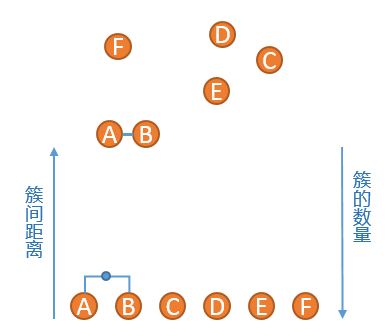3、重复步骤2、发现{C}和{D}的距离最短，连接之，然后是簇{C,D}和簇{E}距离最短，依次类推，直到最后只剩下一个簇，得到如下所示的示意图：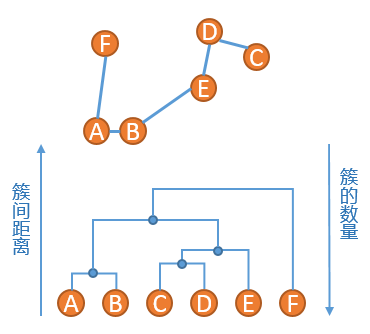4、此时原始数据的聚类关系是按照层次来组织的，选取一个簇间距离的阈值，可以得到一个聚类结果，比如在如下红色虚线的阈值下，数据被划分为两个簇：簇{A，B，C，D，E}和簇{F}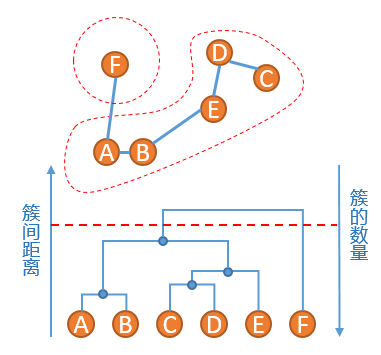Agglomerative聚类算法的优点是能够根据需要在不同的尺度上展示对应的聚类结果，缺点同Hierarchical K-means算法一样，一旦两个距离相近的点被划分到不同的簇，之后也不再可能被聚类到同一个簇，即无法撤销先前步骤的工作。另外，Agglomerative性能较低，并且因为聚类层次信息需要存储在内存中，内存消耗大，不适用于大量级的数据聚类。

Cluster 之间的距离衡量

 方法 定义 Single-link $D(C_i, C_j) = \min_{x \in C_i, y \in C_j } d(x, y)$ Complete-link $D(C_i, C_j) = \max_{x \in C_i, y \in C_j } d(x, y)$ UPGMA $D(C_i, C_j) = \frac{1}{|C_i||C_j|} \sum_ { x \in C_i, y \in C_j} d(x, y)$ WPGMA 省略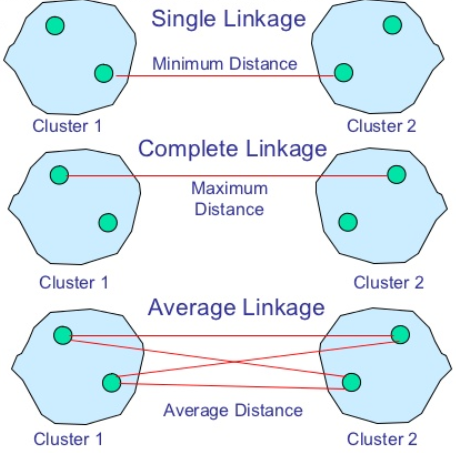Centroid/UPGMC方法给每一个cluster 计算一个质心，两个 cluster 之间的距离即为对应的两个质心之间的距离，一般计算方法如下：

$$D_{UPGMC}(C_i,C_j) = \frac{1}{|C_i||C_j|} \sum_{x \in C_i, y \in C_j}d(x, y) – \frac{1}{2{|C_i |}^2}\sum_{x, y\in C_i} d(x,y) – \frac{1}{2{|{C_j}|}^2} \sum_{x,y \in C_j}d(x, y)$$

Median/WPGMC 方法为每个 cluster 计算质心时，引入了权重。

Ward方法提出的动机是最小化每次合并时的信息损失，具体地，其对每一个 cluster 定义了一个 ESS （Error Sum of Squares）量作为衡量信息损失的准则，cluster C 的 ESS 定义如下：

${ESS}(C) = \sum_{x \in C} (x- m_x)^{T}(x – m_x)$

常用Agglomerative算法

Lance-Williams 方法

$$D(C_k, C_i \cup C_j) = \alpha_i D(C_k, C_i) + \alpha_j D(C_k, C_j) + \beta D(C_i, C_j) + \gamma | D(C_k, C_i) – D(C_k, C_j)|$$

 方法 参数$\alpha_i$ 参数$\alpha_j$ 参数$\beta$ 参数$\gamma$ Single-link 1/2 1/2 0 -1/2 Complete-link 1/2 1/2 0 1/2 UPGMA $n_i/(n_i + n_j)$ $n_j/(n_i + n_j)$ 0 0 WPGMA 1/2 1/2 0 0 UPGMC $n_i/(n_i + n_j)$ $n_j/(n_i + n_j)$ $n_{i}n_{j}/(n_i + n_j)^2$ 0 WPGMC 1/2 1/2 1/4 0 Ward $(n_k + n_i)/(n_i + n_j + n_k)$ $(n_k + n_j)/(n_i + n_j + n_k)$ $n_k/(n_i + n_j + n_k)$ 0

Naive 算法

1. 初始时每个样本为一个 cluster，计算距离矩阵 D，其中元素$D_{ij}$为样本点$x_i$和$x_j$之间的距离
2. 遍历距离矩阵 D，找出其中的最小距离（对角线上的除外），并由此得到拥有最小距离的两个 cluster 的编号，将这两个 cluster 合并为一个新的 cluster 并依据 Lance-Williams 方法更新距离矩阵 D （删除这两个 cluster 对应的行和列，并把由新 cluster 所算出来的距离向量插入 D 中），存储本次合并的相关信息
3. 重复 2 的过程，直至最终只剩下一个 cluster。

利用 Scipy 实现层次聚类

1、生成测试数据

from sklearn.datasets import make_blobs
import matplotlib.pyplot as plt

centers = [[1, 1], [-1, -1], [1, -1]]
X, labels_true = make_blobs(n_samples=750, centers=centers, cluster_std=0.4, random_state=0)
plt.figure(figsize=(10, 8))
plt.scatter(X[:, 0], X[:, 1], c='b')
plt.show()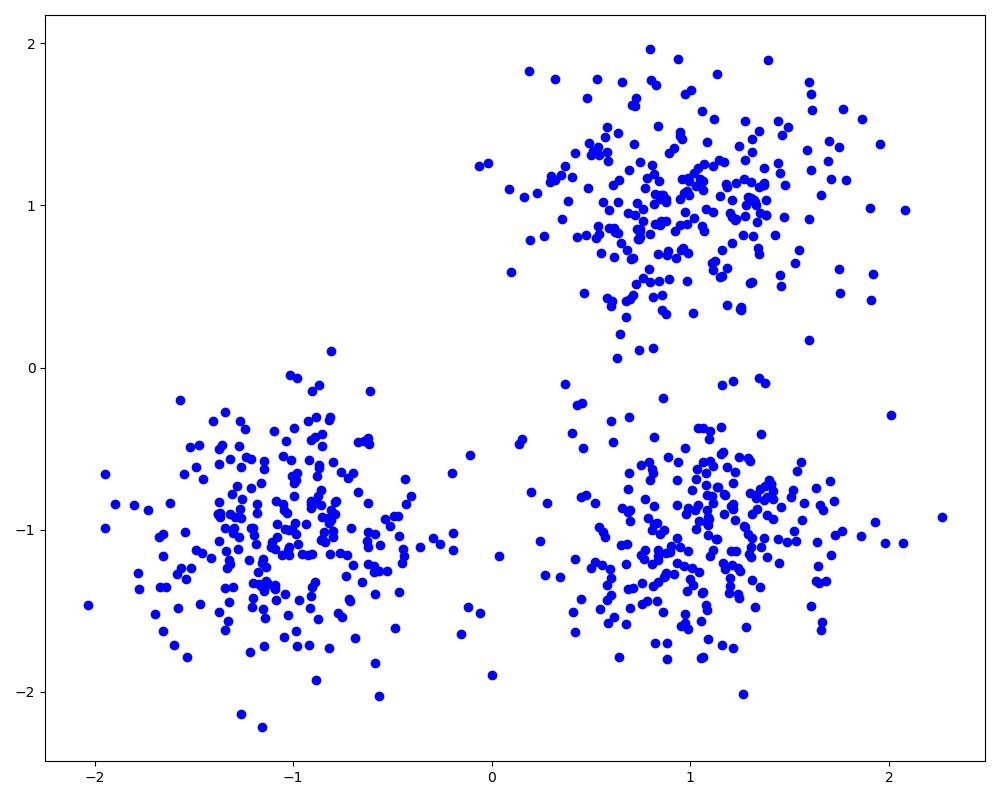print(Z.shape)
print(Z[: 5])

linkage 函数的返回值 Z 为一个维度(n-1)*4的矩阵，记录的是层次聚类每一次的合并信息，里面的 4 个值分别对应合并的两个cluster的序号、两个cluster之间的距离以及本次合并后产生的新的 cluster 所包含的样本点的个数。

3、画出树形图

SciPy 中给出了根据层次聚类的结果 Z 绘制树形图的函数dendrogram，我们由此画出本次实验中的最后 20 次的合并过程。

from scipy.cluster.hierarchy import dendrogram

plt.figure(figsize=(10, 8))
dendrogram(Z, truncate_mode='lastp', p=20, show_leaf_counts=False, leaf_rotation=90, leaf_font_size=15,
show_contracted=True)
plt.show()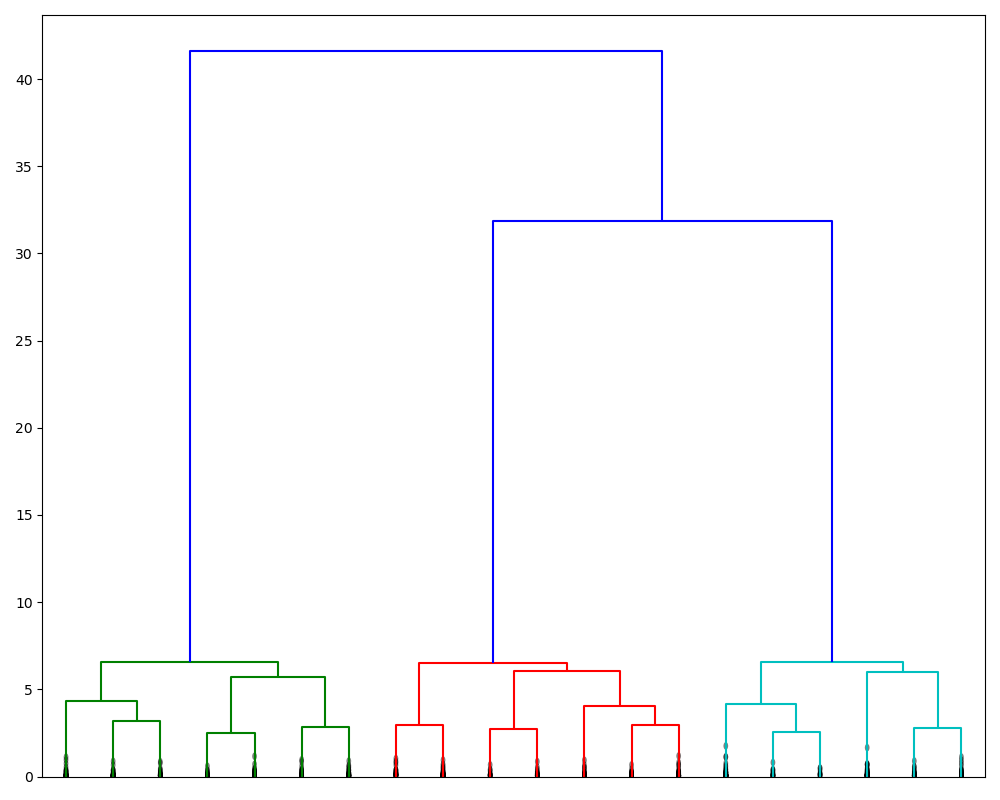4、获取聚类结果

from scipy.cluster.hierarchy import fcluster

# 根据临界距离返回聚类结果
d = 15
labels_1 = fcluster(Z, t=d, criterion='distance')
print(labels_1[: 100])  # 打印聚类结果
print(len(set(labels_1)))  # 看看在该临界距离下有几个 cluster

# 根据聚类数目返回聚类结果
k = 3
labels_2 = fcluster(Z, t=k, criterion='maxclust')
print(labels_2[: 100])
list(labels_1) == list(labels_2)  # 看看两种不同维度下得到的聚类结果是否一致

# 聚类的结果可视化，相同的类的样本点用同一种颜色表示
plt.figure(figsize=(10, 8))
plt.scatter(X[:, 0], X[:, 1], c=labels_2, cmap='prism')
plt.show()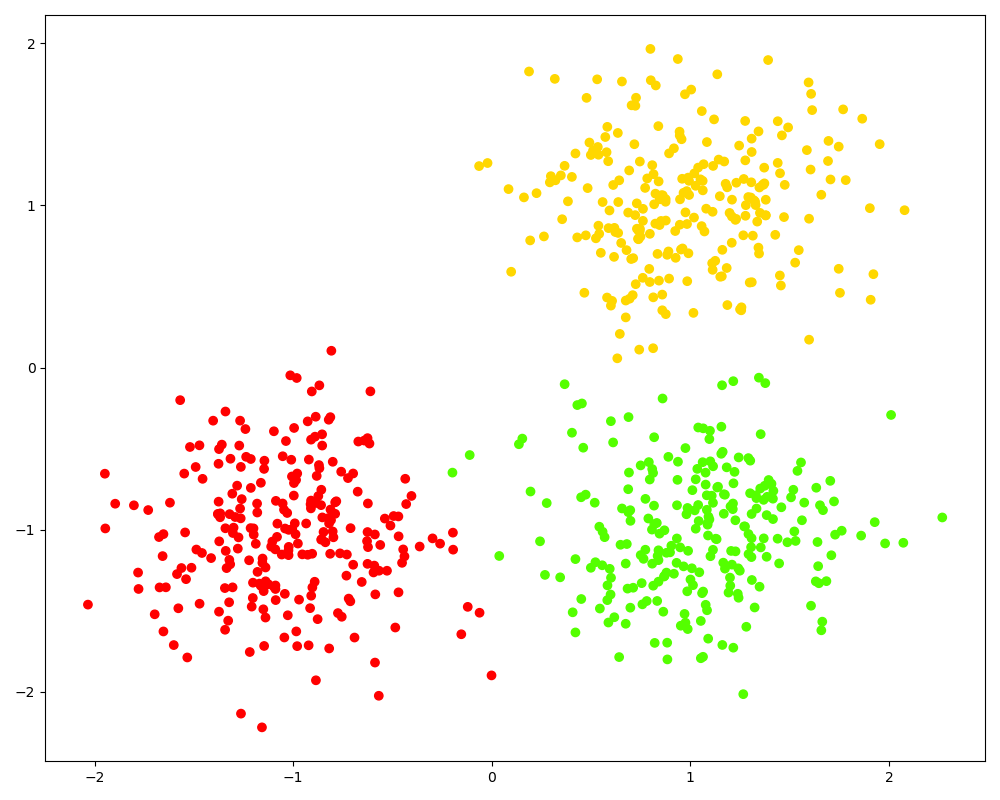5、比较不同方法下的聚类结果

from time import time
import numpy as np
from sklearn.datasets import make_blobs
import matplotlib.pyplot as plt

# 生成样本点
centers = [[1, 1], [-1, -1], [1, -1]]
X, labels = make_blobs(n_samples=750, centers=centers,
cluster_std=0.4, random_state=0)

# 可视化聚类结果
def plot_clustering(X, labels, title=None):
plt.scatter(X[:, 0], X[:, 1], c=labels, cmap='prism')
if title is not None:
plt.title(title, size=17)
plt.axis('off')
plt.tight_layout()

# 进行 Agglomerative 层次聚类
linkage_method_list = ['single', 'complete', 'average', 'ward']

plt.figure(figsize=(10, 8))
ncols, nrows = 2, int(np.ceil(len(linkage_method_list) / 2))
plt.subplots(nrows=nrows, ncols=ncols)
start_time = time()
labels_pred = fcluster(Z, t=3, criterion='maxclust')
print('time used: %.3f seconds' % (time() - start_time))
plt.subplot(nrows, ncols, i + 1)
plt.show()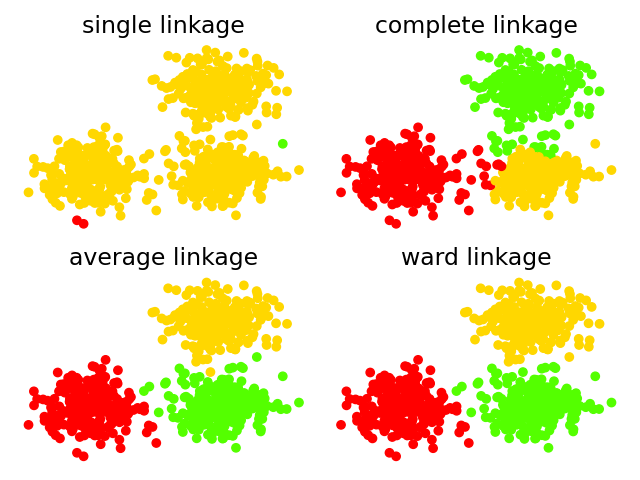method single:
time used: 0.010 seconds
method complete:
time used: 0.021 seconds
method average:
time used: 0.027 seconds
method ward:
time used: 0.021 seconds

利用 Sklearn 实现层次聚类

from sklearn.datasets import make_blobs
from sklearn.cluster import AgglomerativeClustering
import matplotlib.pyplot as plt

# 生成样本点
centers = [[1, 1], [-1, -1], [1, -1]]
X, labels = make_blobs(n_samples=750, centers=centers,
cluster_std=0.4, random_state=0)